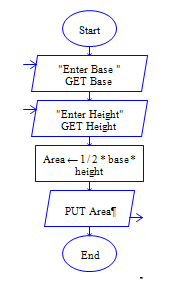# Java Program to find area of Right-angled triangle

Get length and width of right angled triangle and find area of right angled triangle

Sample Input 1:

4 5

Sample Output 1:

10

#### Flow Chart Design#### Program or Solution

``` import java.util.*; class RightATri { public static void main(String args[]) { float length,breadth,area; Scanner sc=new Scanner(System.in); System.out.println("Get the length and breadth of Right Angled Triangle and find area of it."); System.out.println("Enter The length:"); length=sc.nextInt(); System.out.println("Enter The breadth"); breadth=sc.nextInt(); area=(length*breadth)/2; System.out.println("The Output 1 Is:\n"+area); } } ```

#### Program Explanation

Get length and breadth of a right angled triangle (using scanner class) Calculate area by dividing product of length and breadth by 2 (area = length * breadth/2) print area (using system.out.println)